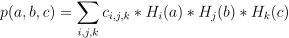/NumPy 1.17

# numpy.polynomial.hermite_e.hermemulx

`numpy.polynomial.hermite_e.hermemulx(c)` [source]

Multiply a Hermite series by x.

Multiply the Hermite series `c` by x, where x is the independent variable.

Parameters: `c : array_like` 1-D array of Hermite series coefficients ordered from low to high. `out : ndarray` Array representing the result of the multiplication.

#### Notes

The multiplication uses the recursion relationship for Hermite polynomials in the formxP_i(x) = (P_{i + 1}(x) + iP_{i - 1}(x)))

#### Examples

```>>> from numpy.polynomial.hermite_e import hermemulx
>>> hermemulx([1, 2, 3])
array([2.,  7.,  2.,  3.])
```